### An R package for Bayesian structural equation modeling.

The package allows Bayesian analysis for particular cases of structural equation models (SEMs) based on rstan integration. Examples in get started and exploring bsem include confirmatory factor analysis and confirmatory SEM. The full SEM model (outer and inner models), enables the evaluation of user-defined latent variables along with the analysis of established linear relationships among the latent scores.

## Install

Please install remotes package and devtools before installing:

``````install.packages("remotes")
install.packages("devtools")``````

Due to R 4.0 chain info is not working properly. As discussed here a possible fix is to get the under development version with:

``remotes::install_github("stan-dev/rstan", ref = "develop", subdir = "rstan/rstan")``

Afterwards, you can install bsem using:

``devtools::install_github("rvpanaro/bsem", dependencies = TRUE)``

## Basic working example

• Get some simulated data.
``dt <- bsem::simdata()``
• Fit your SEM model
``````semfit <- bsem::sem(
data = dt\$data,
blocks = dt\$blocks,
paths = dt\$paths,
exogenous = dt\$exogenous,
signals = dt\$signals,
iter = 2000,
warmup = 1000,
chains = 4
)``````
• Print the outcome:
``print(semfit) ``
• Get posterior summary statistics:
``summary(semfit)``
• Plot a diagram with the relatioships between variables:
``plot(semfit)``

## Access to ShinyApp

``bsem::runShiny()``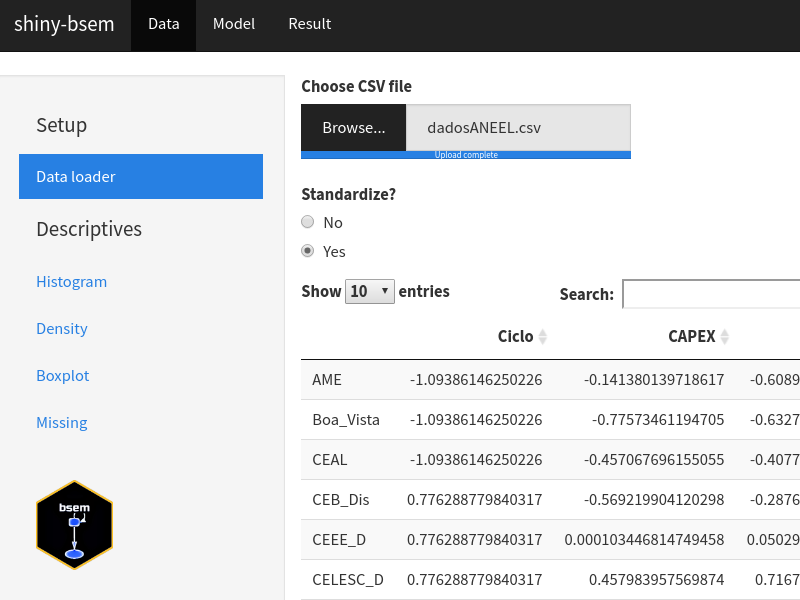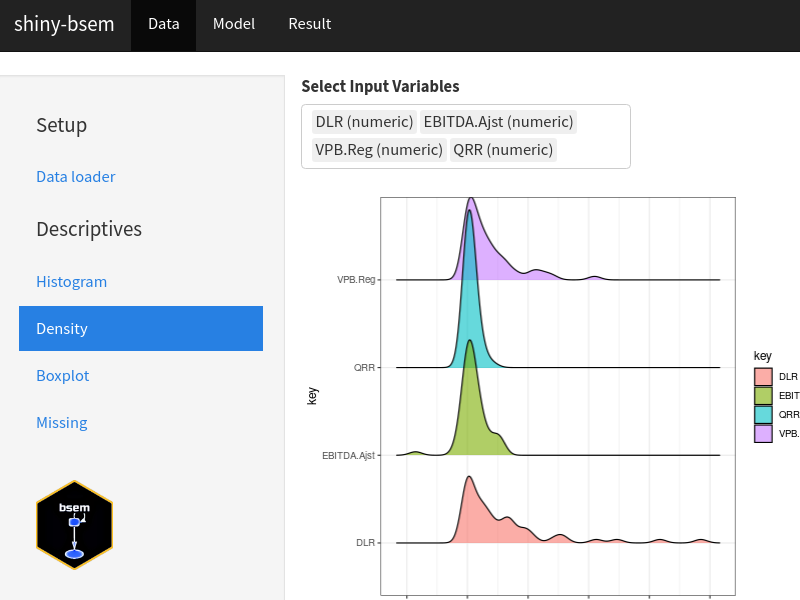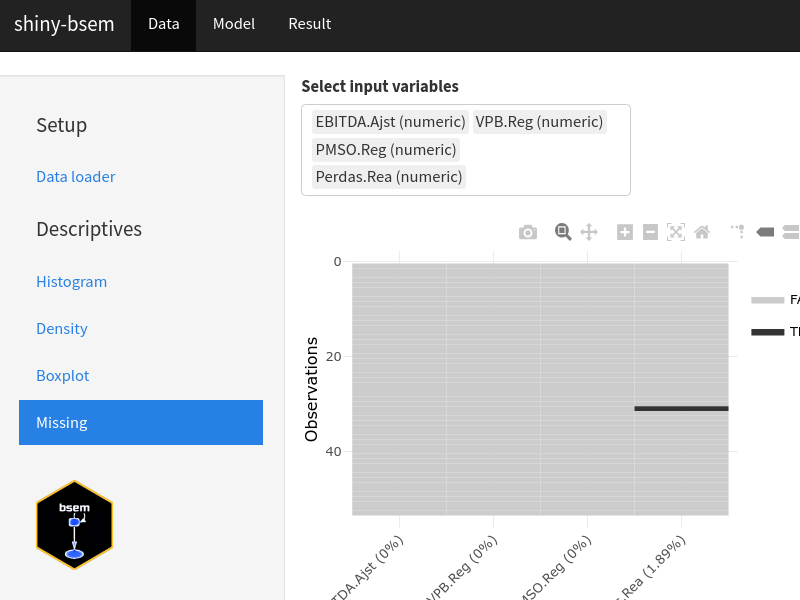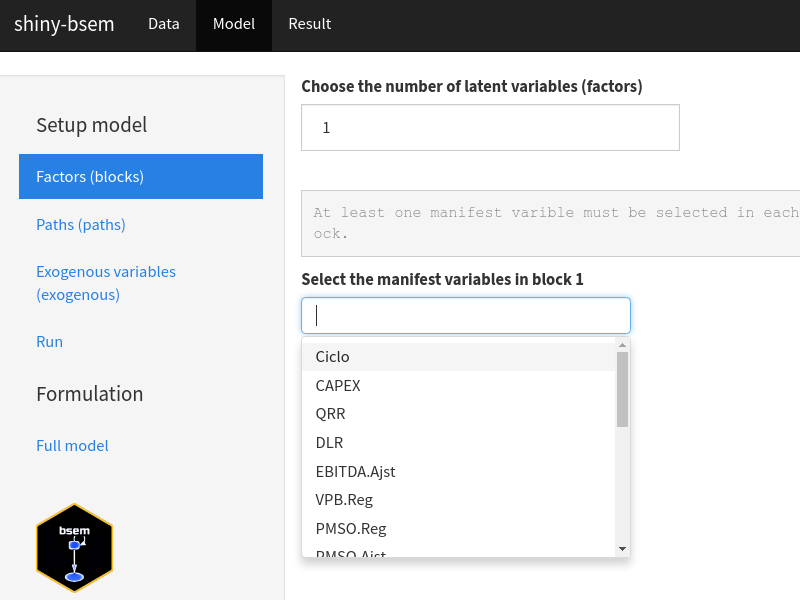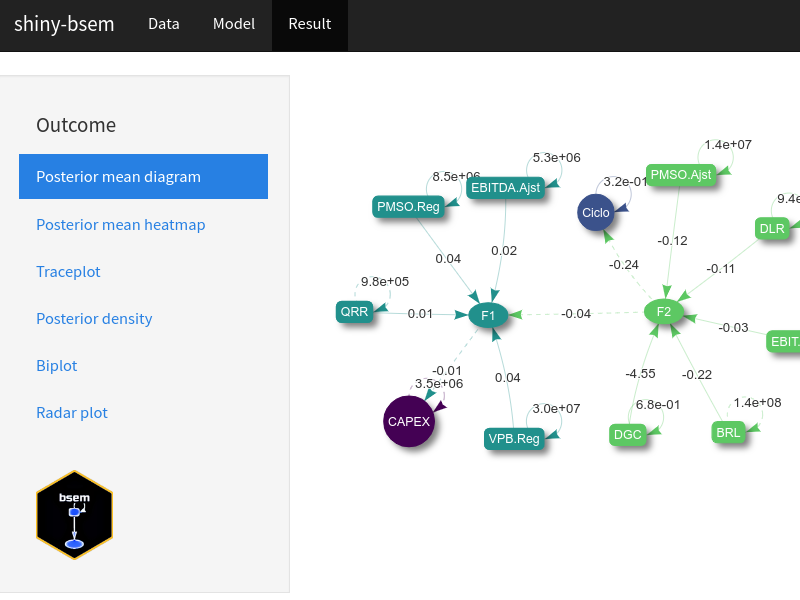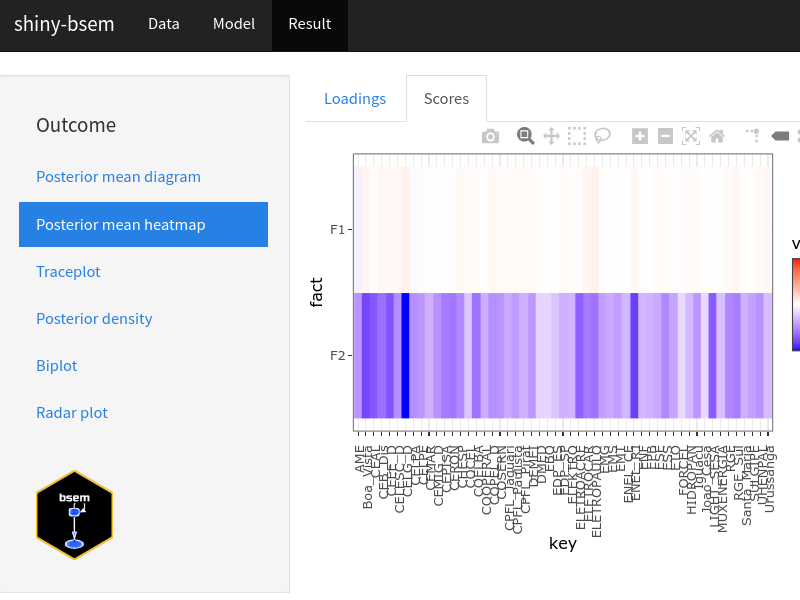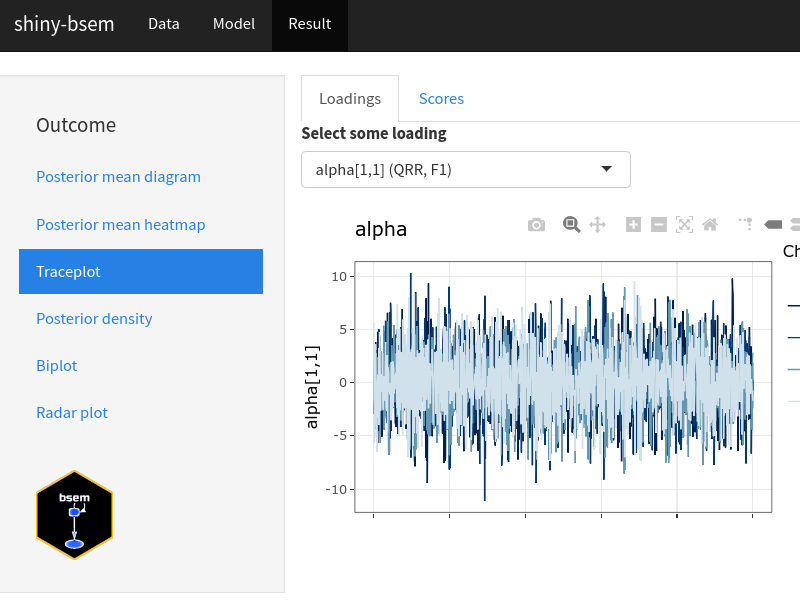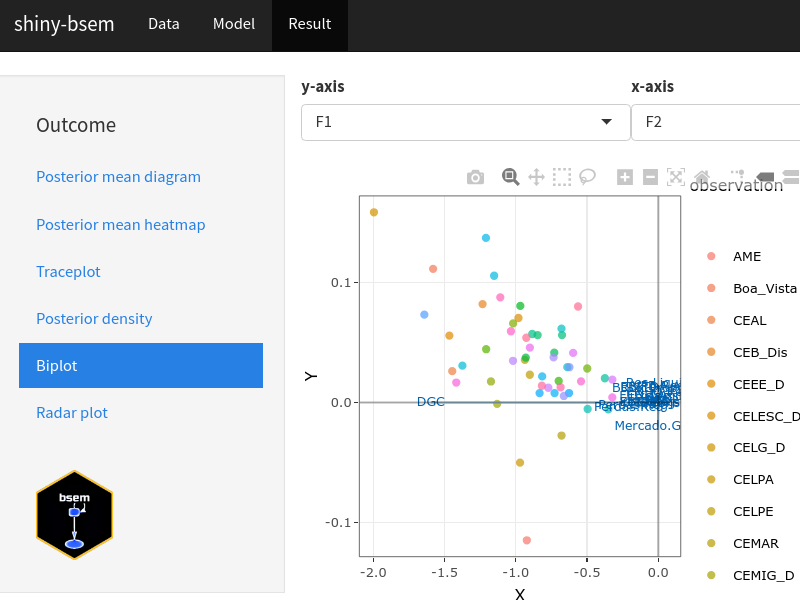## Future work and improvements

Feel free to collaborate with me or to open an issue.